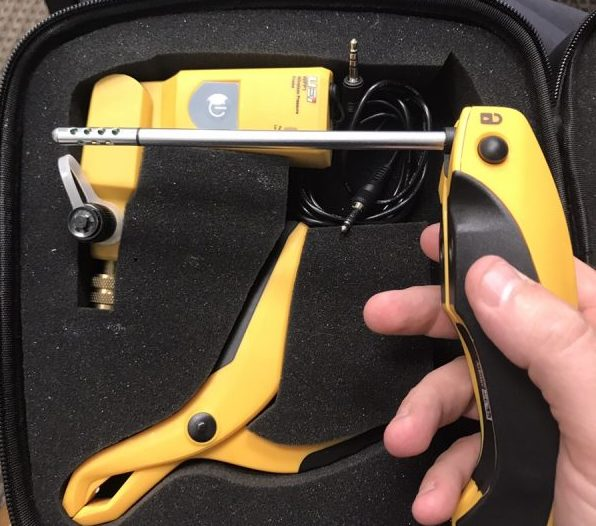## What is Enthalpy?Enthalpy is easy… it’s just a state function that depends only on the prevailing equilibrium state identified by the system’s internal energy, pressure, and volume. It is an extensive quantity. Simple.

Like most things, the scientific definition is as clear as mud. In HVAC/R we use enthalpy measurement to come up with the total heat change in a fluid, whether it’s refrigerant, water or air.

That total change in heat content or enthalpy change is called Delta H (ΔH) which is just another way of saying “total heat split” and it is generally measured in BTU/lb in the US.

In air, we need to use probes that measure humidity and temperature like the HUB2 probes shown above or the Testo 605i probes in order to calculate the enthalpy of the air. Air has both the energy associated with the temperature of the air as well as the latent heat stored in the water vapor.UEI HUB Screenshot

If you want to use the Δto calculate the total heat added or removed from the air you would then use this formula to calculate BTUs of heat added or removed from the air.

Total Heat = (H1-H2) x 4.5 x CFM

In the case above it would be

Total Heat = (29.68 – 22.77) x 4.5 x 730 (CFM we measured)

so

29.68 – 22.77 = 6.91 Δ

6.91 x 4.5 x 730 = 22,699.35 BTU/hr

This total air enthalpy change is a required part of calculating total system capacity and is a pretty simple thing to understand.

Don’t confuse ΔH (Total Heat Change) with ΔT (Temperature Difference). ΔH includes both latent and sensible heat and is a measure of heat quantity in BTU/lb while ΔT only calculates temperature difference and isn’t converted to BTUs at all.

— Bryan

This site uses Akismet to reduce spam. Learn how your comment data is processed.

Scroll to top
Translate »

### Daily Tech Tip

Get the (near) daily Tech Tip email right in your inbox!